blog

# Long Gamma and Short Gamma Explained

#### Options Trading 101 - The Ultimate Beginners Guide To Options

As Seen On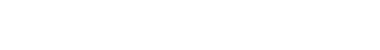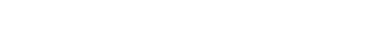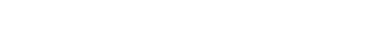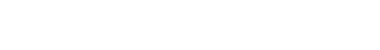by Gavin in Blog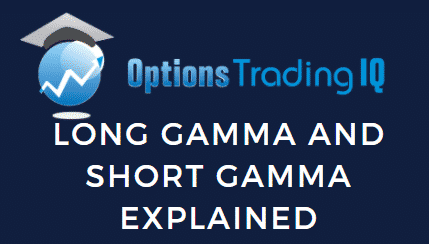What’s better, being long gamma or short gamma? Today, we will answer that question, plus a lot more.

## Introduction

Option gamma indicates how an is expected to change when the underlying stock price changes.

Gamma is one of the least understood greeks so I want to try and help you gain a much better understanding today.

One of the main things to understand about gamma is that the exposure and risks increase the closer you get to expiry. You can read about that in detail here.

That’s an important concept, but let’s look at the difference between long gamma and short gamma.

## Long Gamma Vs Short Gamma

Long gamma (also called positive gamma) indicates that the trade’s delta will increase as the stock rises and decreases as the stock falls. Long gamma traders want the stock to continue trending in the same direction

Short gamma (also called negative gamma) indicates that the trade’s delta will decrease as the stock rises and increases as the stock falls. Short gamma traders want the stock to revert back towards the starting price.

Long straddles have positive gamma and the trader want the stock to keep moving in the one direction (either up or down)

Short straddles have negative gamma and as the stock moves, the trader wants the stock to revert back to where it started.

We’ll look at some specific examples shortly, but let’s use a theoretical example first to illustrate the concept.

## Long Gamma Example

Let’s use a simple example of a long put and a long call.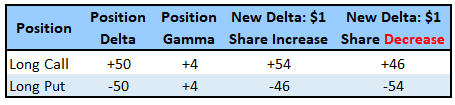The long call has positive delta and positive gamma (long gamma). As the stock price rises, the delta increases. It like a snowball effect, the position exposure grows in the same direction as the stock.

If the stock decreases, the delta exposure also decreases.

The long put has negative delta and positive gamma (long gamma). As the stock price decreases, the delta becomes more negative. In this case the position exposure also grows in the same direction as the stock.

In both these cases the exposure is getting bigger as the position is moving for the trader.

## Short Gamma Example

Now we’ll look at a short call and a short put.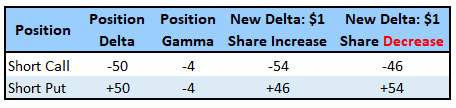The short call has negative delta and negative gamma (short gamma). As the stock price rises, the delta becomes more negative indicating that the trader wants the position to revert back to where it started.

If the stock decreases, the delta exposure becomes less negative.

The short put has positive delta and negative gamma (short gamma). As the stock price decreases, the delta becomes more positive indicating that the trader wants the stock to rally back up.

In both these cases the exposure is getting bigger as the position is moving against the trader.

## Real Life Examples

It’s always good to look at some real-life examples to help solidify the concepts

Date: July 27, 2020

Current Price: \$323.27

Buy 10 SPY Sept 18th, 324 call @ \$9.75
Buy 10 SPY Sept 18th, 324 put @ \$11.16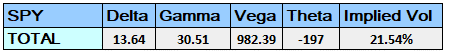Notice that the trade has positive gamma of 30.51.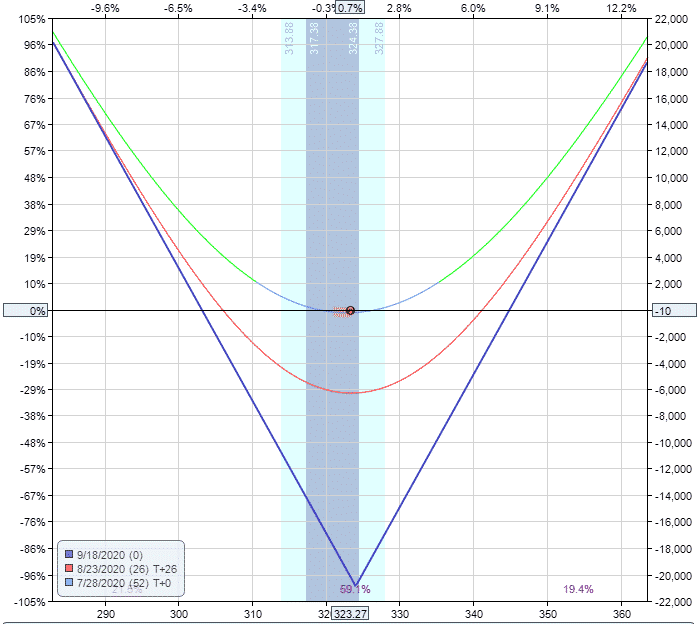Below you can see how the trade changes over the first 10 days of the trade.

Notice that as the prices rises, delta becomes more positive just as we would expect with a long gamma trade.

I’ve also included two columns on the right hand side after gamma. The first column takes the previous day’s ending delta and adds the sum of gamma and the price change.

Notice that the delta moves very much inline with what we would expect. It’s never going to be perfect because there are a lot of moving parts and delta will also be impacted by changes in vega and theta.

But, you can see that our calculated delta is very close to the actual delta.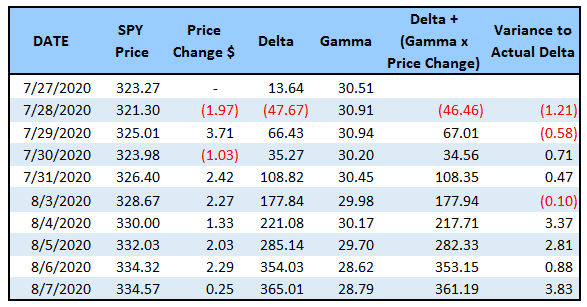Let’s look at a short gamma example.

Date: April 1, 2020

Current Price: \$240.89

Sell 5 AAPL May 15th, 245 call @ \$17.32
Sell 5 AAPL May 15th, 245 put @ \$21.90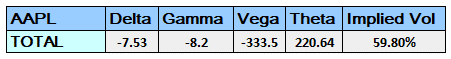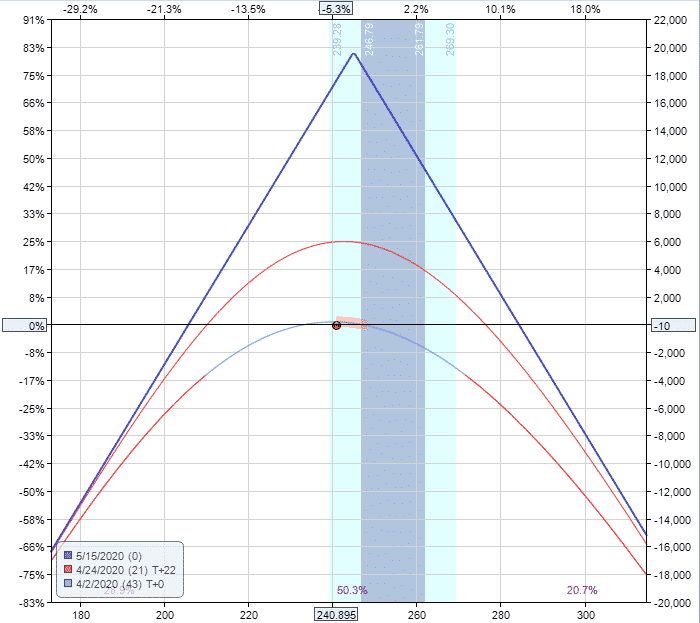Here we are seeing the exact opposite of the previous example as you would expect. As AAPL stock rises, the delta becomes more and more negative.

This is because the trader wants the stock to drop back down into the profit zone.

Interestingly in this example, the manual calculation of delta isn’t as accurate.

I think it’s because volatility was so high at the time and moving around a lot, from a high of 59.80% to a low of 38.41%.

It’s safe to assume that other factors were also impacted the change in delta in addition to the gamma, but gamma still would have been the major factor.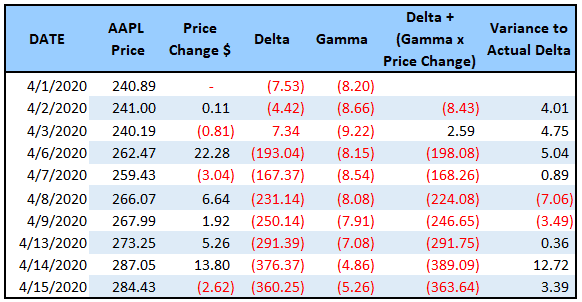## Summary

While gamma is one of the lesser known greeks and arguably least important of the “big 4”, it’s important to have at least a basic understanding of the difference between long gamma and short gamma.

The easiest way to remember gamma is this:

Gains on long gamma trades will grow exponentially as the stock continues to trend. Long gamma traders want trending stocks.

Losses on short gamma trades will grow exponentially as the stock continues to trend. Short gamma traders want stocks to stay in a tight range.

I hope you enjoyed this tutorial.